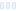# Energy Formula Hype Sauce

## G Fuel

Main info:

Energy Formula Hype Sauce
G Fuel
1 scoop
15 Calories
3 g
0 g
0 g

0 g
0 mg
0 g
79.0 mg
0 g
0 g

Nutrition Facts
For a Serving Size of (g)
How many calories are in Energy Formula Hype Sauce? Amount of calories in Energy Formula Hype Sauce: Calories Calories from Fat (%)
% Daily Value *
How much fat is in Energy Formula Hype Sauce? Amount of fat in Energy Formula Hype Sauce: Total Fat
How much sodium is in Energy Formula Hype Sauce? Amount of sodium in Energy Formula Hype Sauce: Sodium
How many carbs are in Energy Formula Hype Sauce? Amount of carbs in Energy Formula Hype Sauce: Carbohydrates
How many net carbs are in Energy Formula Hype Sauce? Amount of net carbs in Energy Formula Hype Sauce: Net carbs
How much sugar is in Energy Formula Hype Sauce? Amount of sugar in Energy Formula Hype Sauce: Sugar
How much protein is in Energy Formula Hype Sauce? Amount of protein in Energy Formula Hype Sauce: Protein
Vitamins and minerals
How much Calcium is in Energy Formula Hype Sauce? Amount of Calcium in Energy Formula Hype Sauce: Calcium
How much Iron is in Energy Formula Hype Sauce? Amount of Iron in Energy Formula Hype Sauce: Iron
Fatty acids
Amino acids
* The Percent Daily Values are based on a 2,000 calorie diet, so your values may change depending on your calorie needs.
Percent calories from...Loading similar foods...
Note: Any items purchased after clicking our Amazon buttons will give us a little referral bonus. If you do click them, thank you!Be cool

- Zen orangeI never skip arm day

- Buff broccoli